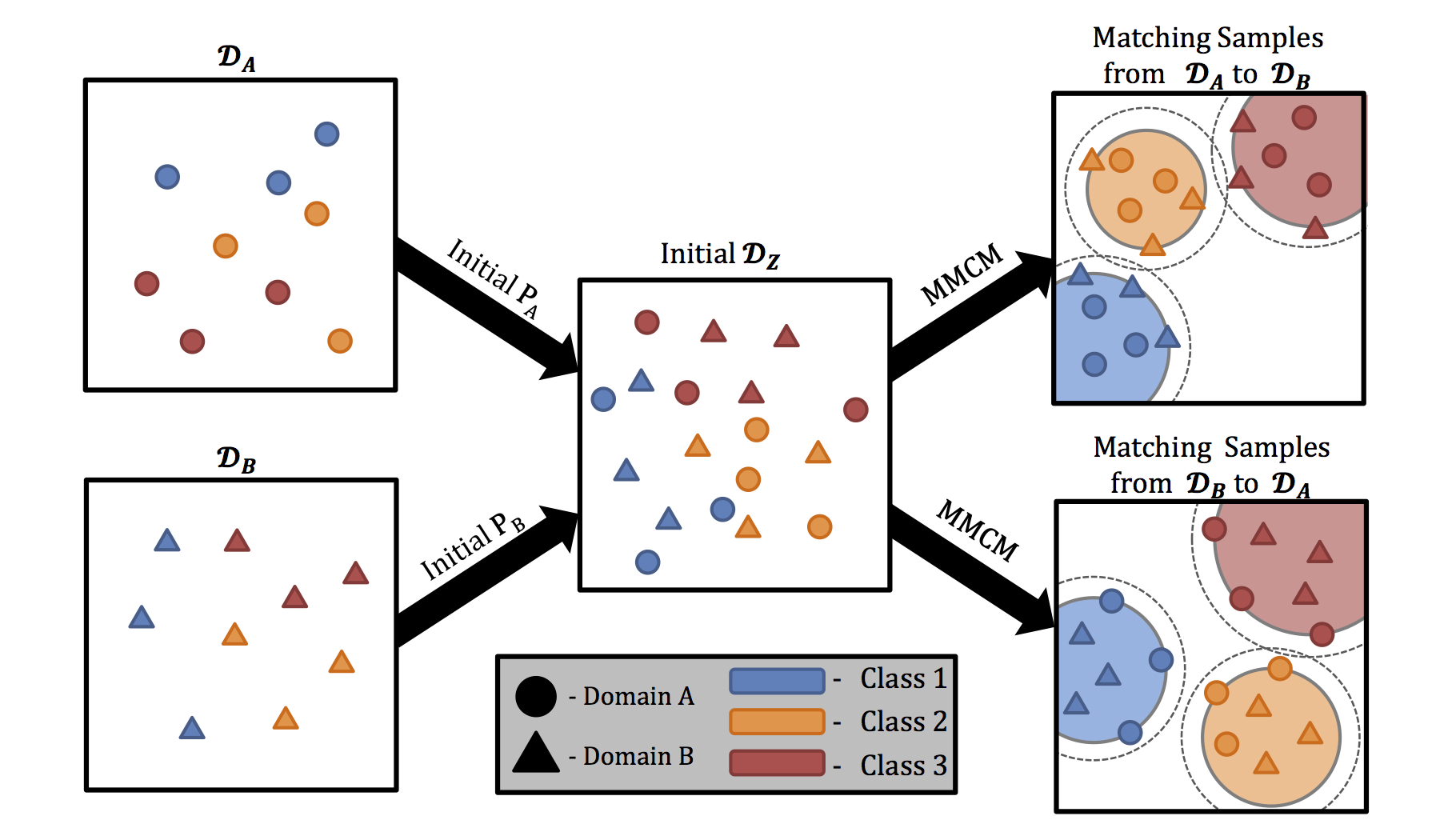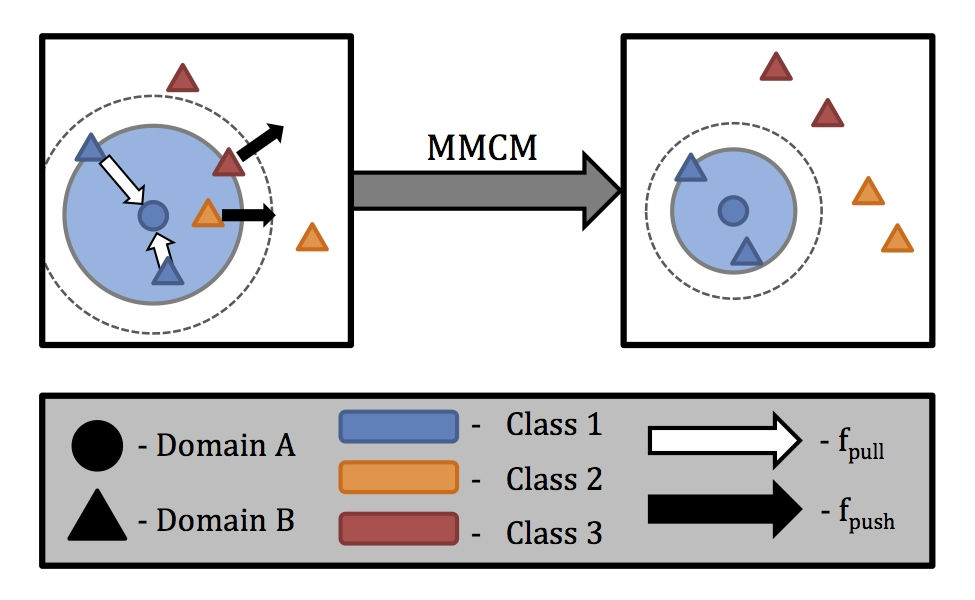Source code
Contributors
Latest commits

### Abstract

Over the past decade, fueled by cheaper storage and availability of ever increasing computational resources, there has been an explosive increase in the collection of data about the same concept (e.g. face images) from multiple sources and in multiple formats. This leads to pattern matching scenarios across that necessitate the development of learning algorithms that have the ability to learn concepts from diverse sources of data, much like human learning. In this paper, we consider this problem of concept learning from and across multiple sources of data in the context of face recognition under challenging scenarios i.e., we consider situations in the wild where the probe and gallery images are captured under different conditions, thereby treating the probe and gallery images as arising from different domains. We present Maximum-Margin Coupled Mappings (MMCM), a method which learns linear projections to map the data from the different domains into a common latent domain, maximizing the margin of separation between between the intraclass data and the interclass data from different domains. We demonstrate the effectiveness of this technique on multiple face biometric datasets with a variety of cross-domain relationships.

### OverviewOverview of coupled mappings and MMCM. Coupled mappings project data from $$\mathcal{D}_{A}$$ and $$\mathcal{D}_{B}$$ into a common subspace, $$\mathcal{D}_{Z}$$ , where matching can be done between data samples from the different domains. MMCM learns mappings optimized for matching data samples from one domain to the second, but not vice versa (i.e. comparing samples from $$\mathcal{D}_{A}$$ to a gallery from $$\mathcal{D}_{B}$$ , but not samples from $$\mathcal{D}_{B}$$ to a gallery from $$\mathcal{D}_{A}$$, or vice versa). MMCM learns coupled mappings such that there is a margin between cross-domain matches and the nearest cross-domain non-matches. The cross-domain match distances define a perimeter around each class, and no cross-domain non-matches enter a margin extending from this perimeter.Illustration showing the objective of the MMCM optimization, and the effect of $$f_{pull}$$ and $$f_{push}$$ (for simplicity, only a single data sample from $$\mathcal{D}_{A}$$ is shown). The large blue circle represents the perimeter defined by the largest match pair distance, and the dashed line shows the margin extending from that perimeter. Initially, cross-domain matches are far from the data sample from $$\mathcal{D}_A$$, and cross-domain non-matches are within boundary of the margin. The $$f_{pull}$$ term brings together the match pairs, and $$f_{push}$$ moves the intruding non-matches outside of the boundary of the margin, while non-matches outside of the margin are not explicitly acted upon. The result is that the data samples from Class 1 are closer together, and non-matches are now outside of the margin around Class 1.

### Contributions

• Present a new coupled mapping formulation called Maximum-Margin Coupled Mappings (MMCM).
• Combines the common subspace learning principle of coupled mapping techniques and the margin maximizing properties of single domain large margin nearest neighbor.
• Extensive evaluation of MMCM under different cross-domain matching scenarios.